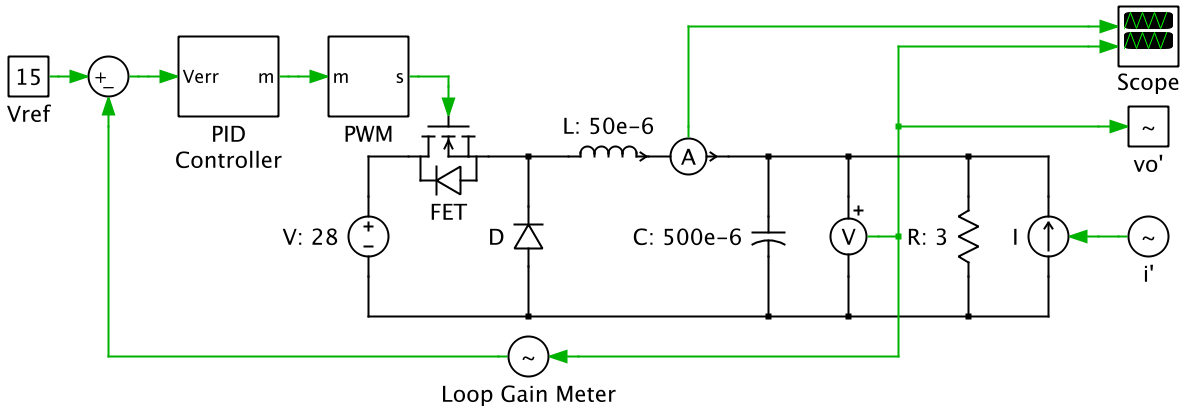# Voltage mode (PWM) control

For a buck converter, I am trying to understand how the appropriate signal is sent to the PWM block to control the duty cycle of the FET. When the output voltage is equal to your reference voltage (15V in picture), the error is 0. So does that mean the PID controller somehow outputs a signal of 0.5357 assuming a 0V to 1V sawtooth signal to compare to for the comparator to generate the PWM?

Because with a buck converter: Vout = D*Vin, so Vout/Vin = 28V/15V = 0.5357 = D.• If the error is zero the controller neither increases nor decreases the pulse width... Rather it holds it at that pulse width till an error voltage is detected then slews the width in the right direction. Mar 9, 2017 at 3:13